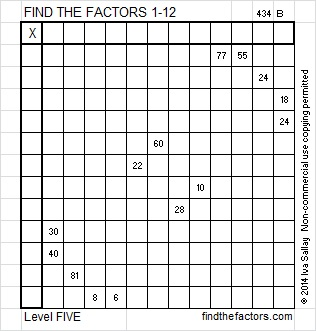# 217 and Level 5

• 217 is a composite number.
• Prime factorization: 217 = 7 x 31
• The exponents in the prime factorization are 1 and 1. Adding one to each and multiplying we get (1 + 1)(1 + 1) = 2 x 2 = 4. Therefore 217 has 4 factors.
• Factors of 217: 1, 7, 31, 217
• Factor pairs: 217 = 1 x 217 or 7 x 31
• Since 217 has no square factors, its square root cannot be simplified. √217 ≈ 14.731Excel file of puzzles and previous week’s factor solutions: 12 Factors 2014-08-25This site uses Akismet to reduce spam. Learn how your comment data is processed.